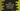# Java program to find pairs with a given sum in an array## Java program to find pairs with a given sum in an array :

### Problem :

One array is given with unsorted numbers. Find number of pairs of elements the sum of which is equal to a given value :

### Solution :

1. We will scan the array two times.
2. Create one empty HashMap first
3. On first iteration, check if any key same as the element of the array exist in the hashmap
4. If not exist, add it with value as 1
5. For array {1,2,3,4,5,4,3}_ , the map(key,value) will look like _1 : 1, 2 : 1, 3 : 2 ,4 : 2, 5 : 1
6. On second iteration, check if any key same as the (sum - element) exist in the map. if exist, increment count by one and delete the key element.
7. In the above example, let’s say sum is 6. So, for firs value 1, sum - value i.e. 6-1 = 5 , key as 5 exist in the map. We will increment the count by one and delete the element with key 5. If the key element is deleted, we will not count two times i.e. for {1,5} and for {5,1}.Since 5 is deleted after {1,5}, we will not get {5,1} pair again.
8. We are also using ‘StringJoiner’ to store the paired elements.
9. StringJoiner values are printed before final count is returned.

### Example program :

``````import java.util.HashMap;
import java.util.StringJoiner;

public class Test {

static int elements[] = new int[]{1, 5, 6, 5, 3, 7, 4, -1, 11};

static int findCount(int[] elementsArray, int sum) {

StringJoiner joiner = new StringJoiner(",");
joiner.setEmptyValue("No pairs fount"); //if stringJoiner is empty , print this msg

//hashmap to store count of each elements appearance
HashMap<Integer, Integer> map = new HashMap<>();

//final count
int count = 0;

for (int i = 0; i < elementsArray.length; i++) {
if (!map.containsKey(elementsArray[i])) {
//if the map doesn't contain the key, initialize it as 1
map.put(elementsArray[i], 1);
}
}

for (int i = 0; i < elementsArray.length; i++) {
if (map.containsKey(elementsArray[i])) {
//difference between sum and the element
int difference = sum - elementsArray[i];

if (map.containsKey(difference)) {
//storing the pairs in a stringjoiner
count += 1;

//removing the key
map.remove(difference);
}
}
}

System.out.println(joiner.toString());
return count;
}

public static void main(String[] args) {
System.out.print("Total pairs " + findCount(elements, 10));
}
}``````

### Output :

``````5:5,4:6,7:3,11:-1
Total pairs 4``````

If you are new to StringJoiner, you can check our ‘StringJoiner’ tutorial here.